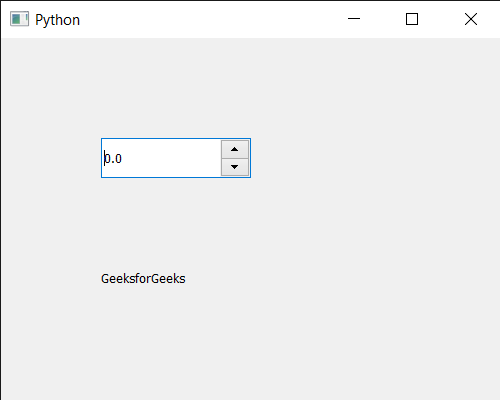# PyQt5 QDoubleSpinBox – Setting Line Edit to it

In this article we will see how we can set the line edit to the QDoubleSpinBox. Line edit is the white space which receives the input and shows the current value of the double spin box, we can change the line edit object any time. Line edit is the main component of the double spin box the default line edit is made such that it receives only float values.

In order to do this we will use `setLineEdit` method with the double spin box object.

Syntax : dd_spin.setLineEdit(lineedit)

Argument : It takes QLineEdit object as argument

Return : It returns None

Below is the implementation

 `# importing libraries ` `from` `PyQt5.QtWidgets ``import` `*`  `from` `PyQt5 ``import` `QtCore, QtGui ` `from` `PyQt5.QtGui ``import` `*`  `from` `PyQt5.QtCore ``import` `*`  `import` `sys ` ` `  ` `  `class` `Window(QMainWindow): ` ` `  `    ``def` `__init__(``self``): ` `        ``super``().__init__() ` ` `  `        ``# setting title ` `        ``self``.setWindowTitle(``"Python "``) ` ` `  `        ``# setting geometry ` `        ``self``.setGeometry(``100``, ``100``, ``500``, ``400``) ` ` `  `        ``# calling method ` `        ``self``.UiComponents() ` ` `  `        ``# showing all the widgets ` `        ``self``.show() ` ` `  `    ``# method for components ` `    ``def` `UiComponents(``self``): ` ` `  `        ``# creating double spin box ` `        ``d_spin ``=` `QDoubleSpinBox(``self``) ` ` `  `        ``# setting geometry to the double spin box ` `        ``d_spin.setGeometry(``100``, ``100``, ``150``, ``40``) ` ` `  `        ``# setting decimal precision ` `        ``d_spin.setDecimals(``1``) ` ` `  `        ``# step type ` `        ``step_type ``=` `QAbstractSpinBox.AdaptiveDecimalStepType ` ` `  `        ``# adaptive step type ` `        ``d_spin.setStepType(step_type) ` ` `  `        ``# line edit ` `        ``lineedit ``=` `QLineEdit(``self``) ` ` `  `        ``# setting line edit to the double spin box ` `        ``d_spin.setLineEdit(lineedit) ` ` `  ` `  `        ``# creating a label ` `        ``label ``=` `QLabel(``"GeeksforGeeks"``, ``self``) ` ` `  `        ``# setting geometry to the label ` `        ``label.setGeometry(``100``, ``200``, ``300``, ``80``) ` ` `  `        ``# making label multi line ` `        ``label.setWordWrap(``True``) ` ` `  ` `  ` `  ` `  ` `  `# create pyqt5 app ` `App ``=` `QApplication(sys.argv) ` ` `  `# create the instance of our Window ` `window ``=` `Window() ` ` `  `# start the app ` `sys.exit(App.``exec``()) `

Output :Attention geek! Strengthen your foundations with the Python Programming Foundation Course and learn the basics.

To begin with, your interview preparations Enhance your Data Structures concepts with the Python DS Course.

My Personal Notes arrow_drop_upCheck out this Author's contributed articles.

If you like GeeksforGeeks and would like to contribute, you can also write an article using contribute.geeksforgeeks.org or mail your article to contribute@geeksforgeeks.org. See your article appearing on the GeeksforGeeks main page and help other Geeks.

Please Improve this article if you find anything incorrect by clicking on the "Improve Article" button below.

Article Tags :

Be the First to upvote.

Please write to us at contribute@geeksforgeeks.org to report any issue with the above content.